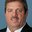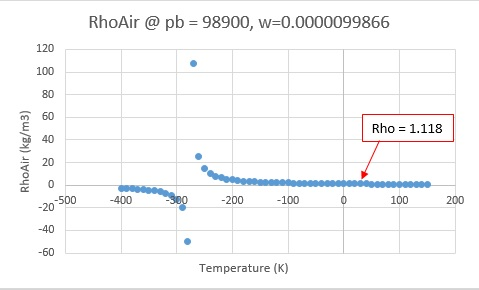Question-and-Answer Resource for the Building Energy Modeling Community
Get started with the Help page

# dx coil pyschrometric errorsDear Forum, Although I have had occasional problems with EnergyPlus giving a fatal error due to DX cooling coil sizing problems, most have been associated with autosized coils. I have a project now which uses 11 different rooftop units, all with DX cooling coils. Three of them are giving me fits (problems). So far, I have:

• disabled the coil below a specified outdoor temp (<= 65F) using an EMS script
• raised the airflow 20% (for improved flow/watt ratio)
• reduced the coil rated capacity by up to 50% (for improved flow/watt ratio)
• disabled the coil completely (availability = always OFF) The only strategy which works is to disable the coil - and that isn't a very good representation of actual performance :)

The current problem coil has these characteristics: - 11,400 cfm VAV fan with minimum flow fraction = 0.7 - 385 MBH / 113 kw rated capacity - single stage coil

The Fatal error looks like: * Severe * PsyRhoAirFnPbTdbW: RhoAir (Density of Air) is calculated <= 0 [-2.69960]. * ~~~ * pb =[98900.00], tdb=[-400.80], w=[9.9866244E-006]. * ~~~ * Routine=Unknown, Environment=STORE 291 - STURGIS (01-01:31-12), at Simulation time=05/16 01:40 - 01:50 * Fatal * Program terminates due to preceding condition.

Can you suggest ways to correct this? Thanks in advance. The rooftop unit uses a digital compressor, which unloads very flexibly. Perhaps I should make the coil multi-stage?

edit retag close merge delete

Sort by » oldest newest most votedThe DX coil model will simulate the coil at full flow and capacity to determine the performance. Then the model will set the outlet conditions based on cycling or constant fan choice. For cycling fan the outlet conditions are full capacity T/w and average air mass flow rate based on PLR. For constant fan the outlet conditions are average T/w based on PLR and whatever flow rate the coil is currently operating. When air flow is lower than expected, very cold outlet temperature is calculated and hence the warnings/fatal. So if the lower air flow rate corresponds to a lower compressor speed, then yes a multi-stage or multi-speed coil model is needed.

At those conditions the empirical model thinks density is negative. The low air flow rate at high compressor capacity is causing very low outlet air temperature and humidity ratio.

rhoair = (pb / (287.0 * (tdb + 273.15) * (1.0 + 1.6077687 * max(w, 1.0e-5))));
pb = barometric pressure
tdb = temperature
w = humidity ratiomore

Clarifying: T/w = "time-weighted" ? If so, all you say makes sense. Thanks! I see a LOT of digital compressors these days, which amount to a variable speed compressor - great flexibility in responding to VAV airflow changes. Do you know of any plans to make an E+ object that is simpler for input than the Coil:Cooling:DX:VariableSpeed?

Outlet conditions T/w = temperature/humidity ratio. No plans as yet for true variable speed. The interpolation at each speed is as close to variable speed as we have at the moment. I believe a component model would be required as is done with the refrigerated racks (which is why no one has taken on the challenge).

Thanks Richard. When I get a minute, I'll suggest it as a new feature

One more thought: How can air density be less than zero? For that matter, how can it even approach zero in atmosphere? Is that a symptom of, or the cause of the fatal error? ..... Last severe error=PsyRhoAirFnPbTdbW: RhoAir (Density of Air) is calculated <= 0 [-8.86462].

I see it... For some reason, E+ thinks the air is in outer space and approaching absolute zero temperature. That strikes me as a logic failure somewhere.

* ~~~ * pb =[98633.33], tdb=[-393.82], w=[9.9865111E-006].